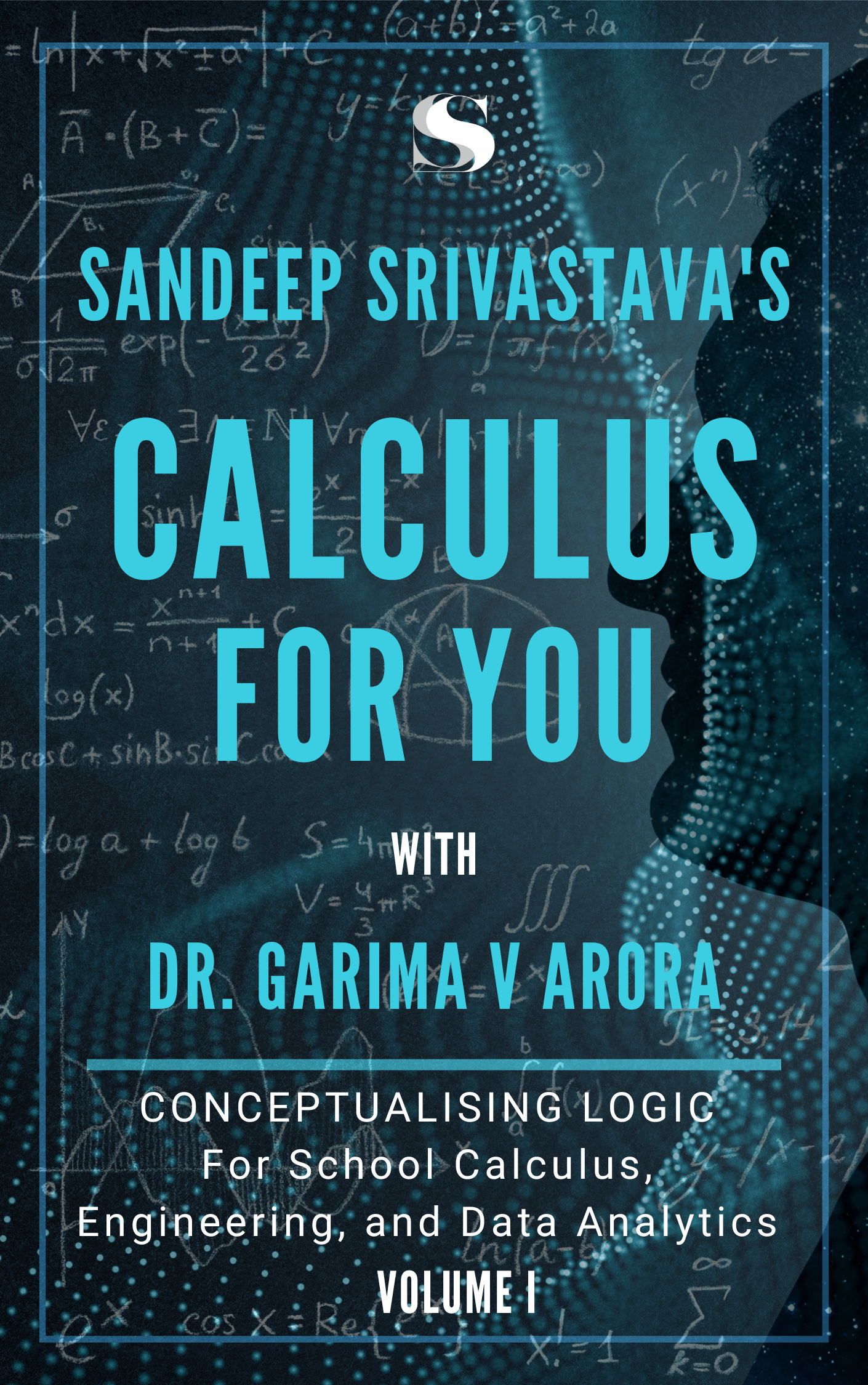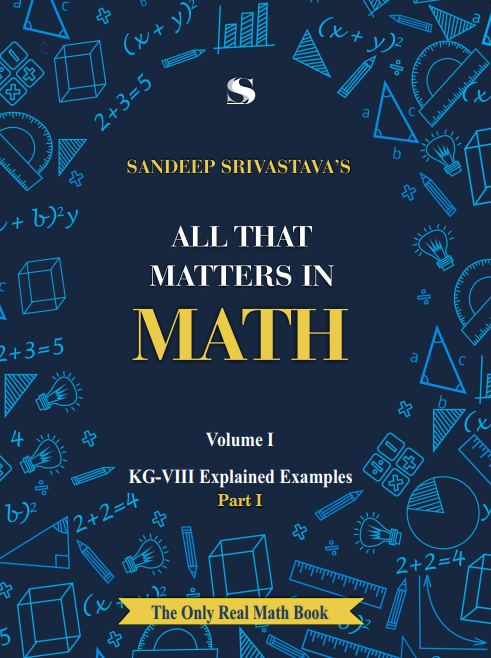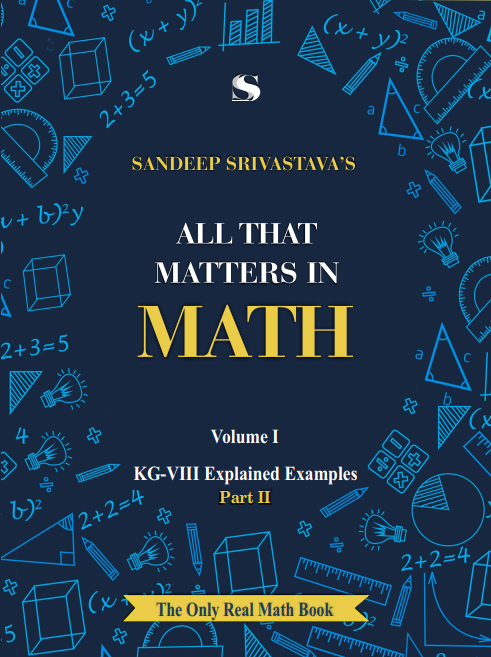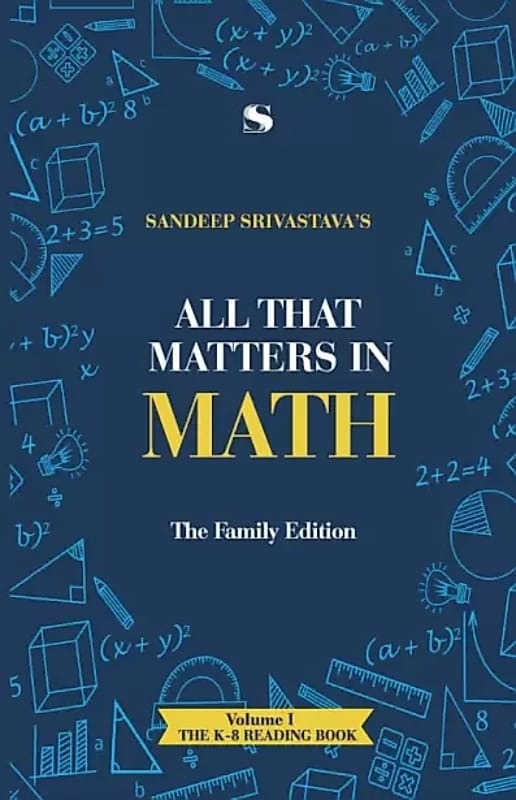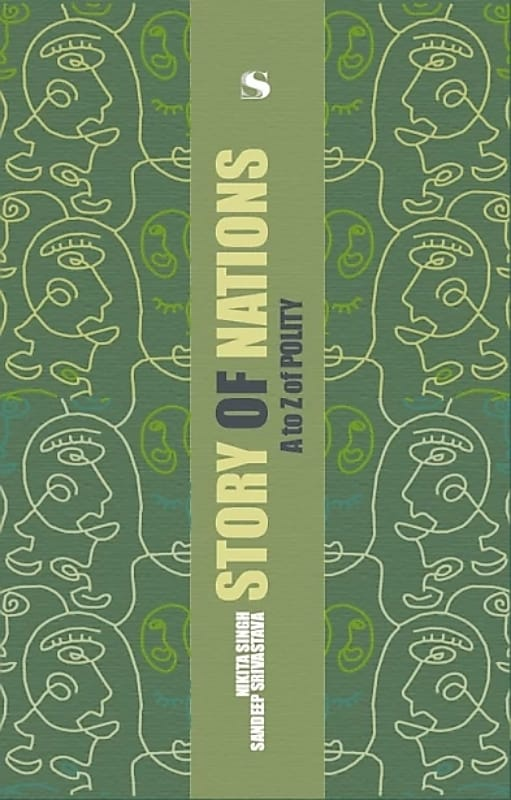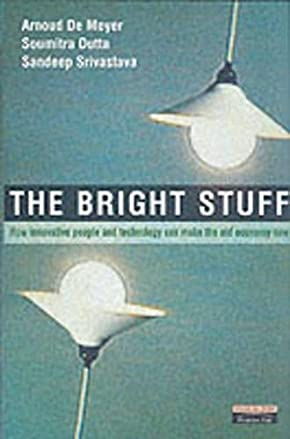## CALCULUS FOR YOU

Zero is of fundamental importance to technology and science; scientific revolution literally waited for the discovery of zero. Calculus is the language of near-zero, instantaneous magnitude of quantities.

The computation of instantaneous values faced three challenges, and calculus specifically addressed them. For example, the physical challenge was addressed using the idea of derivative that avoided such difficult measurements (of near zero time and small distances traversed in that duration, when measuring instantaneous velocity).

The idea of limit addressed the mathematical challenge of using rate for instantaneous values (as such, rate gives average values), and the conceptual challenges of meaningful and valid near zero quantities were addressed using the idea of continuity.
Of course, the masterstroke came in the form of using functions for computations.

## LATEST OFFERINGS